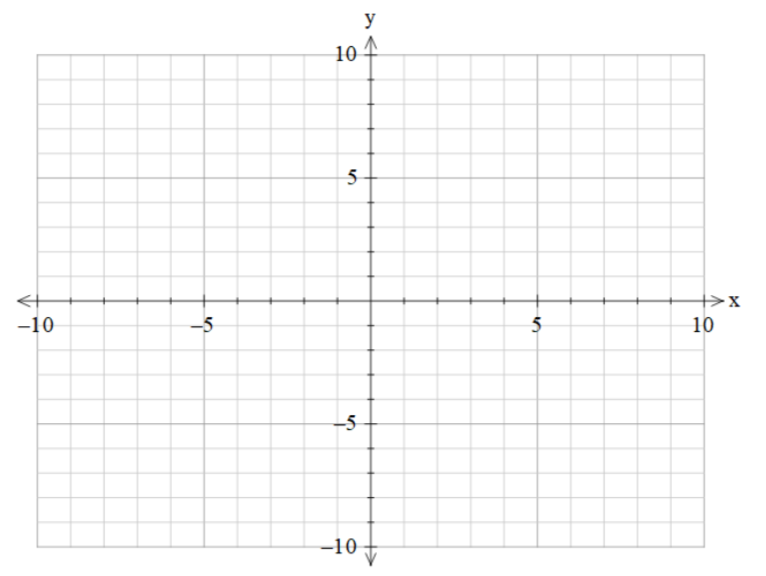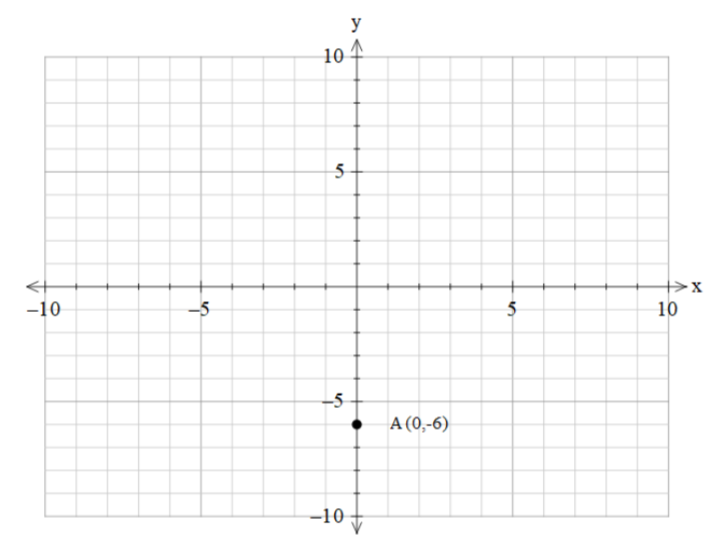QuestionAnswers

# The point (0,-6) lies on (a) y – axis (+ve)(b) x – axis(c) x – axis (-ve)(d) y- axisVerified
129k+ views
Hint: In order to solve this problem, we need to know the orientation of all axis. The horizontal axis is often called the x-axis and the vertical axis is called the y-axis. The upper region is called the + y-axis and the lower part is called as -ve y-axis. The leftmost part is called as -ve x-axis and the rightmost part is called +ve x-axis.

Complete step-by-step solution:
We are given a point and we need to find on which axis it lies on.
If anyone of the coordinates is zero then the coordinate lies on either of the axes.
Now, we can see that the ‘x’-coordinate of the point is zero.
So, we can conclude that it does lie on one of the axes.
The axes divide the quadrant into 4 regions also dividing the axes into 4 sections.
The four sections are +ve x-axis, -ve x-axis, +ve y-axis, -ve y-axis.
The horizontal axis is often called the x-axis and the vertical axis is called the y-axis.
The upper region is called the + y-axis and the lower part is called as -ve y-axis.
The leftmost part is called as -ve x-axis and the rightmost part is called +ve x-axis.
Let's get to know the axis with the help of the graph.Now, let's plot the point that we are given.
The point that we are given is (0,-6).
As the x-coordinate is zero we can say the point lies on the y-axis.
This is because both the axis intersect at the origin so all the point who has x coordinate zero lies on the y-axis.
Now, we need to find whether it lies in the positive y-axis or negative y-axis.
For that, we need to look at the ‘y’-coordinate.
As we can see that the sign of the y coordinate is negative, so it must lie on the negative y-axis.
We can show that through the diagram also.Hence, the correct option is (d).

Note: In this problem, we need to understand that if x coordinate is zero then the point lies on the y-axis, and if y coordinate is zero then the point lies on the x-axis. Also, the point which is non zero always gives whether the point lies on the positive axis or negative axis.